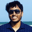Related Tags

community creator

# Submatrix Sum QueriesYash Kumar

This is a competitive programming style question, so let’s define the problem statement with sample input-output and input constraints.

## Problem statement

Given a $N \times M$ matrix consisting of integers, answer $Q$ queries on the matrix of type $(x1, y1, x2, y2)$; i.e., sum the elements in the submatrix with top left corner $(x1, y1)$ and bottom right corner $(x2, y2)$.

### Input format

The first line of input consists of two space-separated integers, $N$ and $M$ $(1 \leq N,M \leq 1000)$.

The next $N$ lines contain M space-separated integers that each represent the matrix.

The next line contains a single integer $Q$ that represents the number of queries $(1 \leq Q \leq 10^6)$.

The next $Q$ lines contain four space-separated integers $x1,y1,x2,y2$, respecitvely$(1 \leq x1,x2 \leq N, 1 \leq y1,y2 \leq M)$.

### Output format

For each $x1,y1,x2,y2$, print a single integer, i.e., the sum of the sub-matrix as described in the problem.

## Sample

### Input

5 5
1 4 7 3 2
3 4 2 5 9
6 1 6 2 1
2 5 8 7 6
3 6 9 8 3
2
2 2 3 5
3 2 5 4


### Output

30
52


## Explanation

For query $1$ -> $x1=2, y1=2, x2=3, y2=5$, the corresponding submatrix is:

The sum of the elements is:

$4 + 2 + 5 + 9 + 1 + 6 + 2 + 1 = 30$

## Brute force

Pretty straightforward. For each query, loop over the submatrix and calculate the sum.

Each query will be answered in $O(N*M)$. The time complexity of the solution will be $O(Q*N*M)$.

With given constraints on $N$ and $M$, we can answer a handful of queries in a few seconds, but definitely not a million queries.

The code for brute force is:

input.txt
5 5
1 4 7 3 2
3 4 2 5 9
6 1 6 2 1
2 5 8 7 6
3 6 9 8 3
2
2 2 3 5
3 2 5 4

## Optimization

We can extend the concept of prefix sums in 1-D arrays to 2 dimensions.

Quick recap. The prefix sum in the 1-D array $A$ is defined as:

$pref[i] = \sum_{k=1}^iA[k]$

Then, the sum of a subarray $A[i...j]$ is $pref[j] - pref[i-1]$.

Let’s define the prefix sum in 2-D, say $pref[i][j]$ is the sum of elements of the submatrix with top left corner as $(1,1)$ and bottom right corner as $(i,j)$, or $pref[i][j] = \sum_{k=1}^i\sum_{l=1}^jA[k][l]$.

pref = A + A + A + A + A + A

Let’s see how we can use the prefix sum matrix to get the sum of any submatrix in $O(1)$ time:

$sum(x1, y1, x2, y2) = pref[x2][y2] - pref[x2][y1- 1] - pref[x1-1][y2] + pref[x1-1][y1-1]$

Let's count how many time each cell is counted in the formula
1 of 10

## Pre-computation

Each query can be answered in $O(1)$ if we have the $pref[]$ array.

The $pref[]$ array can be computed in $O(N*M)$ in a similar way as above. Here is the formula:

$pref[i][j] = pref[i][j-1] + pref[i-1][j] - pref[i-1][j-1] + A[i][j]$

If we iterate the matrix from left to right and top to bottom, at $(i,j)$ we would already have the values at $(i-1,j-1), (i-1,j)$, and $(i,j-1)$. So, calculating the value of $pref[i][j]$ is a constant time operation, i.e., $O(1)$.

Pre-computing the entire $pref[][]$ matrix takes $O(N*M)$ time.

By using $pref[][]$, we can answer each query in $O(1)$.

Thus, the total time complexity is, $O(N*M +Q)$.

## Code

main.cpp
input.txt
#include <iostream>
#include <vector>
#include <utility>
#include <fstream>

using namespace std;

int main() {
ifstream cin("input.txt");

int N, M, Q;
cin >> N >> M;
int A[N][M];
for (int i = 0; i < N; i++)
for (int j = 0; j < M; j++)
cin >> A[i][j];
cin >> Q;

int pref[N][M];
for (int i = 0; i < N ; i++){
for (int j = 0; j < M; j++) {
pref[i][j] = A[i][j];
if (i > 0) pref[i][j] += pref[i-1][j];
if (j > 0) pref[i][j] += pref[i][j-1];
if (i > 0 && j > 0) pref[i][j] -= pref[i-1][j-1];
}
}

while (Q--) {
int x1, y1, x2, y2 ;
cin >> x1 >> y1 >> x2 >> y2;
x1 --; y1--; x2--; y2--;

int sum = pref[x2][y2];
if (y1 > 0) sum -= pref[x2][y1-1];
if (x1 > 0) sum -= pref[x1-1][y2];
if (x1 > 0 and y1 > 0) sum += pref[x1-1][y1-1];

cout << sum << "\n";
}

return 0;
}

RELATED TAGS

community creator

CONTRIBUTORYash Kumar
RELATED COURSES

View all Courses

Keep Exploring

Learn in-demand tech skills in half the time